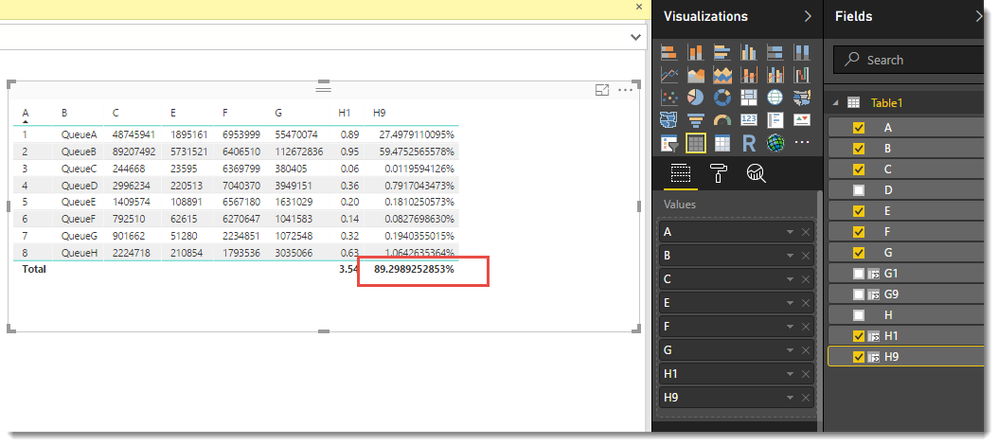cancel
Showing results for
Did you mean:Frequent Visitor

## How do I sum percentages correctly?

Hi,

I have got a request from the business that I support to recreate the following in Power BI.  They are assigning a weight to each row (column G). They then use the sum of the overall weight (G9) to get the overall weighted value in red below (H9).

The calculations are as follows:

column G is =SUM(C2:E2)

column H is =SUM(C2:E2)/SUM(C2:F2)

cell G9 is =SUM(G2:G9)

cell H9 is =(G2*H2/G10)+(G3*H3/G10)+(G4*H4/G10)+(G5*H5/G10)+(G6*H6/G10)+(G7*H7/G10)+(G8*H8/G10)+(G9*H9/G10)

I have tried this numerous ways in Power BI but can't get it to work.  I think I need to get the value in H9 to go row by row somehow?

 A B C D E F G H 1 QueueA 48745941 4828972 1895161 6953999 55470074 0.888600685 2 QueueB 89207492 17733823 5731521 6406510 112672836 0.946199654 3 QueueC 244668 112142 23595 6369799 380405 0.056354593 4 QueueD 2996234 732404 220513 7040370 3949151 0.359356063 5 QueueE 1409574 112564 108891 6567180 1631029 0.198949429 6 QueueF 792510 186458 62615 6270647 1041583 0.14244396 7 QueueG 901662 119606 51280 2234851 1072548 0.324287454 8 QueueH 2224718 599494 210854 1793536 3035066 0.628559985 9 179252692 0.892989253

Thanks!

1 ACCEPTED SOLUTIONMicrosoft

I create the following three calculated columns

```G1 = 'Table1'[C] + 'Table1'[D] + 'Table1'[E]

G9 = CALCULATE(SUM('Table1'[G1]),ALL('Table1'))

H1 = DIVIDE('Table1'[G1] ,  ('Table1'[G1] + 'Table1'[F]))

H9 = 'Table1'[G1] * 'Table1'[H1] / 'Table1'[G9]```Proud to be a Datanaut!

3 REPLIES 3Microsoft

I create the following three calculated columns

```G1 = 'Table1'[C] + 'Table1'[D] + 'Table1'[E]

G9 = CALCULATE(SUM('Table1'[G1]),ALL('Table1'))

H1 = DIVIDE('Table1'[G1] ,  ('Table1'[G1] + 'Table1'[F]))

H9 = 'Table1'[G1] * 'Table1'[H1] / 'Table1'[G9]```Proud to be a Datanaut!Frequent Visitor

Thank you so much for this!!  I'll try it and let you know how it goes.  The actual dataset I'm working with is different from the Excel sheet I got from the business though.  Each queue has many rows associated with it.  So I just need to play with your approach a bit to get it to work.  I'll let you know how it goes.  Thanks again!Frequent Visitor

How would I do this if there are multiple rows per queue?  Do I need to use CALCULATE?  The example I gave had the summed up numbers per queue.  I've tried your approach but I feel there is something up with my grouping.